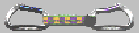# Generation of (Pseudo-)Random Polygonal Data

Besides being a topic of interest of its own, the generation of random polygons has two main areas of application: a) testing the correctness and b) evaluating the CPU-time consumption of algorithms that operate on polygons. For testing the correctness of an algorithm, the goal is to generate a diverse set of input data such that all branches of the algorithm will be executed with a high probability. The same motivation applies to the practical testing of an algorithm's CPU-consumption. Ideally, one would like to test the performance of an algorithm on data of practical relevance. However, it often is next to impossible to obtain a sufficiently large number of practically relevant inputs. Then the second-best choice is to run an algorithm for a reasonably large number of random inputs.

In the mid 90s Thomas Auer and I designed RPG, our Random Polygon Generator. In recent work we renovated RPG and made it compile on current computing platforms. Equally important, we extended its functionality and added several additional stand-alone modules to generate polygonal data with and without holes. Please see the Salzburg Database of Geometric Inputs for both actual datasets as well as links to code available via the Computational Geometry and Applications Laboratory on GitHub.

Related publications:

G. Eder, M. Held, S. Jasonarson, P. Mayer, P. Palfrader (2020):
"Salzburg Database of Polygonal Data: Polygons and Their Generators".
Data in Brief, 31:105984, Aug 2020.

G. Eder, M. Held, S. Jasonarson, P. Mayer, P. Palfrader (2020):
"On Generating Polygons: Introducing the Salzburg Database".
Proc. 36th EuroCG, pp. 75:1-75:7, Würzburg, Germany, March 2020.

Video presentation of the Salzburg Database of Geometric Inputs by Günther Eder (2020).

T. Auer, M. Held (1996):
``Heuristics for the Generation of Random Polygons''.
Proc. 8th Canad. Conf. Computat. Geometry, Carleton University Press, F. Fiala, E. Kranakis, J.-R. Sack (eds.), pp. 38-44; Ottawa, Canada, Aug 12-15, 1996.

The following images show random polygons which were generated by means of RPG. (Click on an image icon in order to see the full-size image. The full-size images have 800x900 pixels.)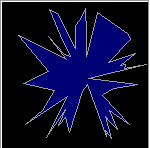This image shows a random star-shaped polygon with 50 vertices.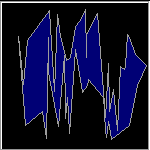This image shows a (truly) random x-monotone polygon with the same set of 50 vertices. (This polygon was constructed according to the algorithm of Chong Zhu, Gopalakrishnan Sundaram, Jack Snoeyink, and Joe Mitchell.)This image shows a "random" simple polygon with the same set of 50 vertices. The polygon was computed using RPG's "2-Opt Moves" heuristic.This image shows a "random" multiply-connected polygon with the same set of 50 vertices. (Holes are shown in cyan.)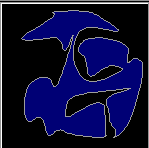This image shows the result of "smoothing" the previous simply-connected polygon three times. (This is a fairly smooth polygon on 400 vertices.)This image shows the result of "smoothing" the previous multiply-connected polygon two times.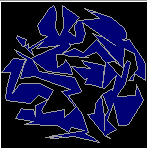This image shows a "random" simple polygon on a set of 200 clustered vertices. The polygon was computed using RPG's "2-Opt Moves" heuristic.This image shows the result of "smoothing" the previous polygon four times.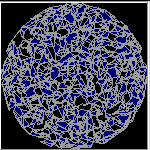This image shows a "random" simple polygon on a set of 10000 clustered vertices. The polygon was computed using RPG's "2-Opt Moves" heuristic.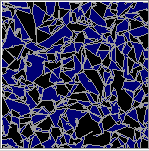This image shows a zoomed view of the previous polygon.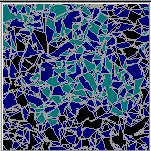This image shows a zoomed view of a multiply-connected polygon on the same set of 10000 clustered vertices. (Holes are shown in cyan.)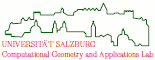file last modified: Wednesday, 26-Aug-2020 08:28:59 CESTCopyright © 2022 Martin Held. All rights reserved.